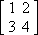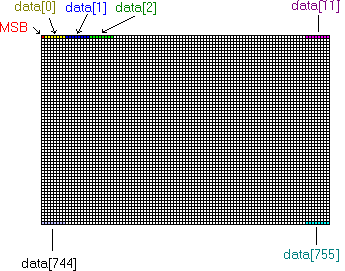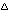TI-83+ Link Protocol Guide v1.0 - Variable formats

## Variable Formats

A TI-83+ variable has two parts: a header and a data section. While the header may be different, the format of the data section never changes whether the variable is in a file, in a transfer packet, or in the calculator's memory. This section describes the format of the data section for most types of variables. The format for the header is described in the packet format and the file format section.

#### Variable Types

There are several types of variables that the TI-83+ uses.

Real Numbers are basic floating-point numbers.
Complex Numbers are basic complex numbers with real and imaginary floating-point components.
Lists are sequences of real or complex numbers used in statistical calculations.
Matrices are two-dimensional matrices with real or complex components.
Y-Variables are functions that evaluate based on the values of one or more variables.
Strings are sequences of text characters.
Graphics Databases (GDB's) are stored sets of graphing functions.
Pictures are stored bitmaps of the graph screen.
Programs are sequences of commands.
Window Settings are stored sets of graph screen range parameters.
Table Settings are stored sets of table screen parameters.

#### Real Numbers

The TI-83+'s representation of a real number has the following format:

Offset Length Description
0 1 byte Flags (see table below)
1 1 bytes Base-10 exponent
2 7 bytes Mantissa

The flags byte has the following format:

1 (02h) If this bit is set, the number is undefined (used for initial sequence values)
2 (04h) If both bits 2 and 3 are set and bit 1 is clear, the number is half of a complex variable.
3 (08h)
6 (40h) Uncertain. Most likely if set, the number has not been modified since the last graph.
7 (80h) Sign bit: If set, the number is negative.

The exponent is a 8-bit unsigned binary number normalized at 80h. The real exponent can be computed by subtracting 80h from the value stored here. Valid exponents are in the range -99 to 99.

The mantissa is stored as a 14-digit unsigned binary-coded-decimal number. The most significant digit is first and the implied decimal point is always between the first two digits. Every nybble (4 bits or half a byte) contains one digit, according to the following table:

Binary Value Decimal Digit

0000

0

0001

1

0010

2

0011

3

0100

4

0101

5

0110

6

0111

7

1000

8

1001

9

#### Complex Numbers

A complex number is represented as a two real numbers, both with flag bits 2 and 3 set. The format is shown below.

Offset Length Description
0 9 bytes A real number describing the "real" component of the complex number.
9 9 bytes A real number describing the "imaginary" component of the complex number.

#### Lists

A list is represented as a sequence of numbers. The format is shown below.

Offset Length Description
0 2 bytes Number of elements in the list
1 n bytes Element values, one by one, first to last. Each element is a 9-byte real number.

#### Matrices

A matrix is represented as a two-dimensional array of numbers. The format is shown below.

Offset Length Description
0 1 byte Number of columns in the matrix (no more than 255)
1 1 byte Number of rows in the matrix (no more than 255)
1 n bytes Element values, one by one (see explanation below). Each element is a 9-byte real number.

The element values are arranged in row definitions from top to bottom. Each row consists of a number of real or complex elements from left to right. Under this scheme,would be ordered (1,2,3,4) in memory.

#### Y-Variables

Y-Variables are represented as a sequence of tokens following a length word. Click here for a table of token definitions.

Offset Length Description
0 2 bytes Number of token bytes in the Y-Variable. Note that some tokens use two bytes.
2 n bytes Tokens, first to last.

#### Strings

Strings are represented in the same way as Y-Variables, but they cannot be evaluated.

#### Graphics Databases (GDB's)

A GDB is a stored database of functions and range parameters. GDB formats are different for each graphing mode (function, parametric, polar or sequence).

A function-mode GDB has the following format:

Offset Length Description
0 2 bytes Length, in bytes, of GDB, minus two.
2 1 byte Unknown
3 1 byte Graphing Mode ID. This byte has a value of 10h for function GDB's.
4 1 byte Mode settings (see mode setting table below)
5 1 byte Unused - has a value of 80h.
6 1 byte Extended mode settings (see extended mode setting table below)
7 9 bytes A real number: Xmin
16 (10h) 9 bytes A real number: Xmax
25 (19h) 9 bytes A real number: Xscl
34 (22h) 9 bytes A real number: Ymin
43 (2Bh) 9 bytes A real number: Ymax
52 (34h) 9 bytes A real number: Yscl
61 (3Dh) 9 bytes A real number: Xres
70 (46h) 10 bytes Ten style bytes, for Y1, Y2, Y3, Y4, Y5, Y6, Y7, Y8, Y9 and Y0, respectively (see style table below).
80 (50h) n bytes Ten functions, for Y1, Y2, Y3, Y4, Y5, Y6, Y7, Y8, Y9 and Y0, respectively (see function table below).

A parametric-mode GDB has the following format:

Offset Length Description
0 2 bytes Length, in bytes, of GDB, minus two.
2 1 byte Unknown - has a value of 0h.
3 1 byte Graphing Mode ID. This byte has a value of 40h for parametric GDB's.
4 1 byte Mode settings (see mode setting table below)
5 1 byte Unused - has a value of 80h.
6 1 byte Extended mode settings (see extended mode setting table below)
7 9 bytes A real number: Xmin
16 (10h) 9 bytes A real number: Xmax
25 (19h) 9 bytes A real number: Xscl
34 (22h) 9 bytes A real number: Ymin
43 (2Bh) 9 bytes A real number: Ymax
52 (34h) 9 bytes A real number: Yscl
61 (3Dh) 9 bytes A real number: Tmin
70 (46h) 9 bytes A real number: Tmax
79 (4Fh) 9 bytes A real number: Tstep
70 (46h) 6 bytes Six style bytes, for X1T/Y1T, X2T/Y2T, X3T/Y3T, X4T/Y4T, X5T/Y5T and X6T/Y6T, respectively (see style table below).
76 (4Ch) n bytes Twelve functions, for X1T, Y1T, X2T, Y2T, X3T, Y3T, X4T, Y4T, X5T, Y5T, X6T and Y6T, respectively (see function table below).

A polar-mode GDB has the following format:

Offset Length Description
0 2 bytes Length, in bytes, of GDB, minus two.
2 1 byte Unknown - has a value of 0h.
3 1 byte Graphing Mode ID. This byte has a value of 20h for polar GDB's.
4 1 byte Mode settings (see mode setting table below)
5 1 byte Unused - has a value of 80h.
6 1 byte Extended mode settings (see extended mode setting table below)
7 9 bytes A real number: Xmin
16 (10h) 9 bytes A real number: Xmax
25 (19h) 9 bytes A real number: Xscl
34 (22h) 9 bytes A real number: Ymin
43 (2Bh) 9 bytes A real number: Ymax
52 (34h) 9 bytes A real number: Yscl
61 (3Dh) 9 bytes A real number:min
70 (46h) 9 bytes A real number:max
79 (4Fh) 9 bytes A real number:step
70 (46h) 6 bytes Six style bytes, for r1, r2, r3, r4, r5 and r6, respectively (see style table below).
76 (4Ch) n bytes Six functions, for r1, r2, r3, r4, r5 and r6, respectively (see function table below).

A sequence-mode GDB has the following format:

Offset Length Description
0 2 bytes Length, in bytes, of GDB, minus two.
2 1 byte Unknown - has a value of 0h.
3 1 byte Graphing Mode ID. This byte has a value of 80h for sequence GDB's.
4 1 byte Mode settings (see mode setting table below)
5 1 byte Sequence mode settings (see sequence mode setting table below)
6 1 byte Extended mode settings (see extended mode setting table below)
7 9 bytes A real number: Xmin
16 (10h) 9 bytes A real number: Xmax
25 (19h) 9 bytes A real number: Xscl
34 (22h) 9 bytes A real number: Ymin
43 (2Bh) 9 bytes A real number: Ymax
52 (34h) 9 bytes A real number: Yscl
61 (3Dh) 9 bytes A real number: PlotStart
70 (46h) 9 bytes A real number: nMax
79 (4Fh) 9 bytes A real number: u(nMin), first element
88 (58h) 9 bytes A real number: v(nMin), first element
97 (61h) 9 bytes A real number: nMin
106 (6Ah) 9 bytes A real number: u(nMin), second element
115 (73h) 9 bytes A real number: v(nMin), second element
124 (7Ch) 9 bytes A real number: w(nMin), first element
133 (85h) 9 bytes A real number: PlotStep
142 (8Eh) 9 bytes A real number: w(nMin), second element
151 (97h) 3 bytes Three style bytes, for u, v and w, respectively (see style table below).
154 (9Ah) n bytes Three functions, for u, v, and w, respectively (see function table below).

The mode setting byte has the following format:

Bit (Mask) Mode if set (1) Mode if clear (0)
0 (01h) Dot Connected
1 (02h) Simul Sequential
2 (04h) GridOn GridOff
3 (08h) PolarGC RectGC
4 (10h) CoordOff CoordOn
5 (20h) AxesOff AxesOn
6 (40h) LabelOn LabelOff
7 (80h) This bit is always clear.

The extended mode setting byte has the following format:

Bit (Mask) Mode if set (1) Mode if clear (0)
0 (01h) ExprOff ExprOn

The sequence mode setting byte has the following format:

Bit (Mask) Mode if set (1) Mode if clear (0)
0 (01h) Web Time, uv, vw or uw
1 (02h) This bit is always clear.
2 (04h) uv Time, web, vw or uw
3 (08h) vw Time, web, uv or uw
4 (10h) uw Time, web, uv or vw
5 (20h) These bits are always clear.
6 (40h)
7 (80h) This bit is always set.
Note: If bits 0-4 are all clear, the graphing mode is "Time."

Each style byte has the following format:
Value Graph Style

0

[solid line]

1

[thick line]

2

3

4

[trace]

5

[animate]

6

[dotted line]

Function definitions have the following format:

Offset Length Description
0 1 byte Flags - For selected functions, this byte is 23h. For deselected or undefined functions, this byte is 03h.
1 n bytes A Y-Variable defining the function. Undefined functions have a token byte length of 0.

#### Pictures

Pictures are 96x63-pixel bitmaps. They have the following format:

Offset Length Description
0 2 bytes Size of picture data (always 2F4h)
2 1008 (3F0h) bytes 1-bit-per-pixel bitmap data, arranged top-to-bottom in rows. Each row is defined left-to-right. (See figure below)

The following figure demonstrates byte ordering of the pixels if the bitmap is in a 756-element array data[]:#### Programs

Programs share the same format as Y-Variables, but are executed rather than evaluated.

TI-BASIC programs are always tokenized, and can be edit-locked or edit-unlocked, depending on the type ID.

Z80 Assembly programs are made up of an ASCII string of hexadecimal digits following the length word. The characters 0-9, A-F, and the hard return character (D6h) are valid. At the end of the program come these three tokenized lines:
:End
:0000
:End

#### Window Settings

Unlike GDB's, window setting formats do not vary with graphing mode. Rather, one window setting structure serves for all graphing modes. An additional window setting structure is saved in the calculator memory for later recall with the name "RclWindow". This special window setting structure does not have the unknown byte that is included in the normal window setting structure.

A normal window setting structure has the following format:

Offset Length Description
0 2 bytes Always has a value of D0h.
2 1 byte Unknown - value is 00h.
3 9 bytes A real number: Xmin
12 (Ch) 9 bytes A real number: Xmax
21 (15h) 9 bytes A real number: Xscl
30 (1Eh) 9 bytes A real number: Ymin
39 (27h) 9 bytes A real number: Ymax
48 (30h) 9 bytes A real number: Yscl
57 (39h) 9 bytes A real number:min
66 (42h) 9 bytes A real number:max
75 (4Bh) 9 bytes A real number:step
84 (54h) 9 bytes A real number: Tmin
93 (5Dh) 9 bytes A real number: Tmax
102 (66h) 9 bytes A real number: Tstep
111 (6Fh) 9 bytes A real number: PlotStart
120 (78h) 9 bytes A real number: nMax
129 (81h) 9 bytes A real number: u(nMin), first element
138 (89h) 9 bytes A real number: v(nMin), first element
147 (93h) 9 bytes A real number: nMin
156 (9Ch) 9 bytes A real number: u(nMin), second element
165 (A5h) 9 bytes A real number: v(nMin), second element
174 (AEh) 9 bytes A real number: w(nMin), first element
183 (B7h) 9 bytes A real number: PlotStep
192 (C0h) 9 bytes A real number: Xres
201 (C9h) 9 bytes A real number: w(nMin), second element

A saved window setting structure has the following format:

Offset Length Description
0 2 bytes Always has a value of CFh.
2 9 bytes A real number: Xmin
11 (Bh) 9 bytes A real number: Xmax
20 (14h) 9 bytes A real number: Xscl
29 (1Dh) 9 bytes A real number: Ymin
38 (26h) 9 bytes A real number: Ymax
47 (2Fh) 9 bytes A real number: Yscl
56 (38h) 9 bytes A real number:min
65 (41h) 9 bytes A real number:max
74 (4Ah) 9 bytes A real number:step
83 (53h) 9 bytes A real number: Tmin
92 (5Ch) 9 bytes A real number: Tmax
101 (65h) 9 bytes A real number: Tstep
110 (6Eh) 9 bytes A real number: PlotStart
119 (77h) 9 bytes A real number: nMax
128 (80h) 9 bytes A real number: u(nMin), first element
137 (88h) 9 bytes A real number: v(nMin), first element
146 (92h) 9 bytes A real number: nMin
155 (9Bh) 9 bytes A real number: u(nMin), second element
164 (A4h) 9 bytes A real number: v(nMin), second element
173 (ADh) 9 bytes A real number: w(nMin), first element
182 (B6h) 9 bytes A real number: PlotStep
191 (BFh) 9 bytes A real number: Xres
200 (C8h) 9 bytes A real number: w(nMin), second element

#### Table Settings

A Table Setting structure stores the table screen parameters. Table settings follow this format:

Offset Length Description
0 2 bytes Always has a value of 12h.
2 9 bytes A real number: TblMin
10 (Ah) 9 bytes A real number:Tbl

Site maintained by Romain Liévin (roms@lpg.ticalc.org) and Tim Singer (tsinger@gladstone.uoregon.edu)